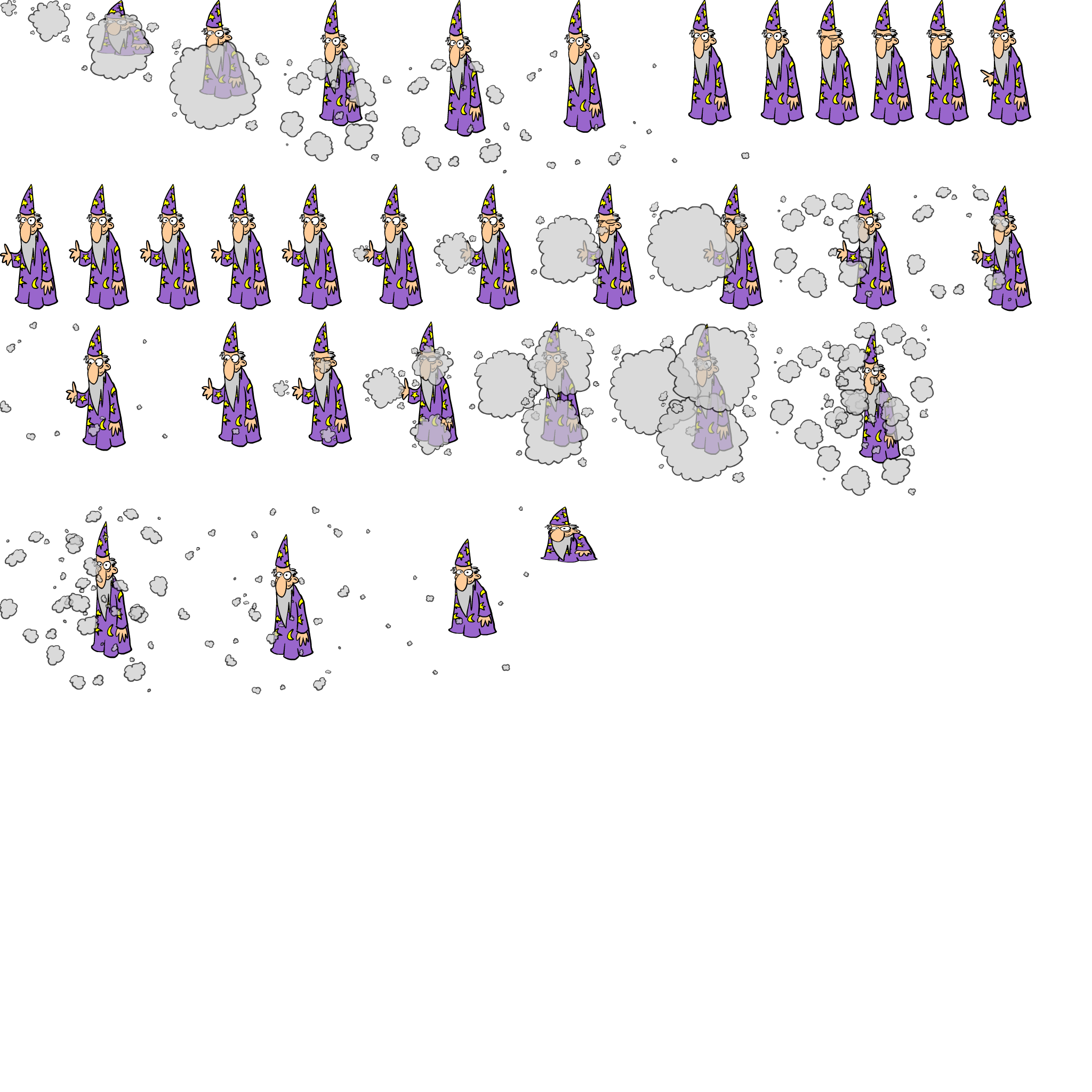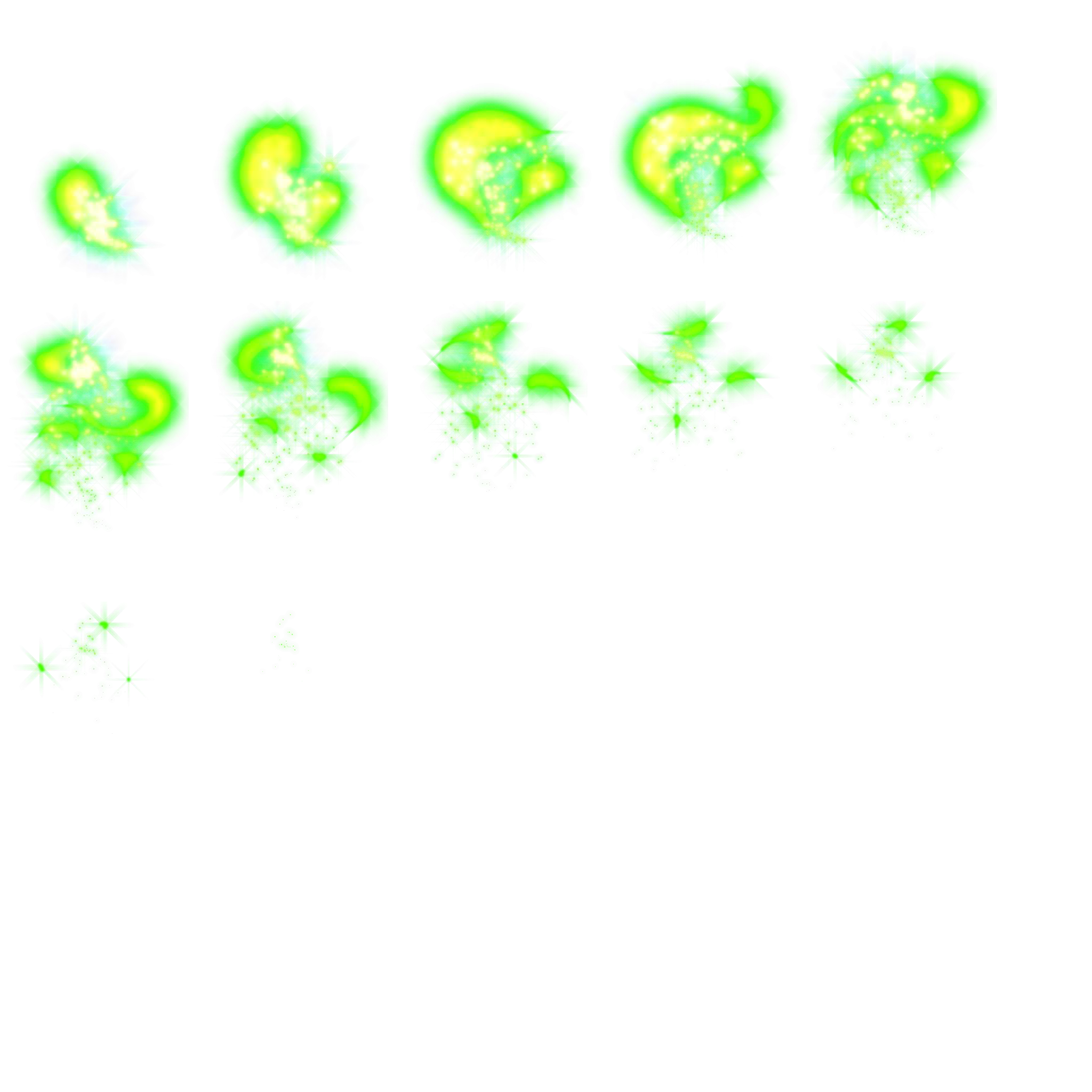i

## Missing Multiplier# Missing Multiplier

Improve your students’ math facts with this magical missing number game.  By selecting the missing multiplier to complete the equation, kids practice their math skills and build their algebraic thinking. Multiplication and division won’t be so tricky after solving these math equations.

### Common Core

Grade 3 » Operations & Algebraic Thinking

CCSS.MATH.CONTENT.3.OA.B.6
Understand division as an unknown-factor problem. For example, find 32 ÷ 8 by finding the number that makes 32 when multiplied by 8.

Visit classplayground.com for Multiplication Printables and Worksheets.

i